Courses

# MRP Work Study And Work Measurement - MCQ Test 2

## 30 Questions MCQ Test Mock Test Series - Mechanical Engineering (ME) for GATE 2020 | MRP Work Study And Work Measurement - MCQ Test 2

Description
This mock test of MRP Work Study And Work Measurement - MCQ Test 2 for Mechanical Engineering helps you for every Mechanical Engineering entrance exam. This contains 30 Multiple Choice Questions for Mechanical Engineering MRP Work Study And Work Measurement - MCQ Test 2 (mcq) to study with solutions a complete question bank. The solved questions answers in this MRP Work Study And Work Measurement - MCQ Test 2 quiz give you a good mix of easy questions and tough questions. Mechanical Engineering students definitely take this MRP Work Study And Work Measurement - MCQ Test 2 exercise for a better result in the exam. You can find other MRP Work Study And Work Measurement - MCQ Test 2 extra questions, long questions & short questions for Mechanical Engineering on EduRev as well by searching above.
QUESTION: 1

Solution:
QUESTION: 2

Solution:
QUESTION: 3

### Assertion (A): Job enrichment increases the job satisfaction of the employee. Reason (R): The jobs of wireman and lineman doing indoor and outdoor works respectively can be integrated for better results.

Solution:
QUESTION: 4

Match List-I (Charts) with List-II (Details) and select the correct answer using the codes given below the lists: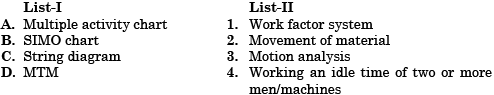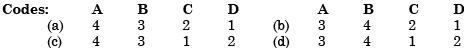Solution:
QUESTION: 5

Procedure of modifying work content to give more meaning and enjoyment to the job by involving employees in planning, organization and control of their work, is termed as

Solution:
QUESTION: 6

Assertion (A): SIMO chart reveals the deficiencies in the motion pattern of the process chart.

Reason (R): SIMO chart and operator processes chart yield the same results.

Solution:
QUESTION: 7

A systematic job improvement sequence will consist of:

(i) Motion Study
(ii) Time Study
(iii) Job Enrichment
(iv) Job Enlargement

An optimal sequence would consist of:

Solution:
QUESTION: 8

Match List-I (Study) with List-II (Related factors) and select the correct answer using the codes given below the lists: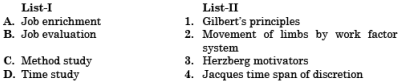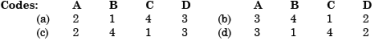Solution:
QUESTION: 9

Which one of the following statements is correct?

Standard time is obtained from normal time by adding the policy allowance and

Solution:

Standard time = Normal time + PDA Allowances

QUESTION: 10

Repetitive fast speed activities can be effectively analyzed by taking photograph at

Solution:
QUESTION: 11

A time standard for a data entry clerk is to be set. A job is rated at 120 percent, it takes 30 seconds to enter each record and the allowances are 15%. What is the normal time?

Solution:
QUESTION: 12

Determination of standard time in complex job system is best done through

Solution:
QUESTION: 13

If in a time study, the observed time is 0.75 min, rating factor = 110% and allowances are 20% of normal time, then what is the standard time?

Solution:

Normal time = Observed Time × Rating Factor = 0.75 ×1.1 = 0.825 min
Standard time = Normal Time × (1 + % age allowance)
= 0.825 × (1 + 0.20) = 0.99 minutes

QUESTION: 14

Standard time is:

Solution:

Normal time = Actual time × Rating factor
Standard time = Normal time + Allowances

QUESTION: 15

In time study, the rating factor is applied to determine

Solution:

In time study, the rating factor is applied to determine merit rating of the worker.

QUESTION: 16

The standard time of an operation has been calculated as 10 min. The worker was rated at 80%. If the relaxation and other allowances were 25%, then the observed time would be:

Solution:

Observed time = (Standard time + Allowances) × Rating of worker
= 10 × (1 + 0.25) × 0.8 = 10

QUESTION: 17

Match List-I (Charts) with List-II (Operations/Information) using the codes given below the lists: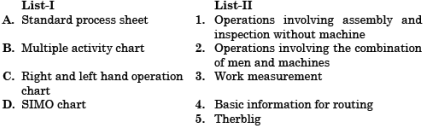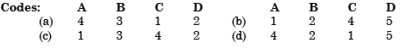Solution:
QUESTION: 18

Consider the following objectives:

1. To train the individual regarding motion economy.
2. To assist in research projects in the field of work study.
3. To help in the collection of Motion Time data.

Solution:

Objectives 1 and 3 are true for Micromotion study.

QUESTION: 19

MTM is a work measurement technique by:

Solution:

MTM (Methods-time measurement) is based on use of standard time for work elements that have been predetermined from long periods of observation and analysis.

QUESTION: 20

Which one of the following is NOT a work measurement technique?

Solution:
QUESTION: 21

Which one of the following is not a technique under Predetermined Motion Time System (PMTS)?

Solution:
QUESTION: 22

Consider the following steps:

1. Method time measurement
2. Work sampling
3. Work factor system.

PMTS (Predetermined motion time systems) in work study would include:

Solution:
QUESTION: 23

Which one of the following statements is not correct?

Solution:
QUESTION: 24

Match List-I with List-II and select the correct answer using the codes given below the lists: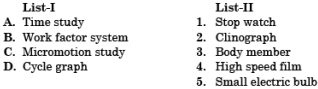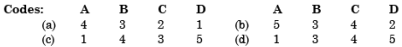Solution:
QUESTION: 25

Work study involves

Solution:
QUESTION: 26

Motions of limbs are through

1. Elbow
2. Finger
3. Hip
4. Shoulder
5. Wrist

What is the correct sequence in descending order of motion in terms of time of fatigue involved?

Solution:
QUESTION: 27

A SIMO chart should be used with

Solution:
QUESTION: 28

Match List-I (Type of Chart) with List-II (Definition) and select the correct answer using the codes given below the lists: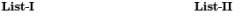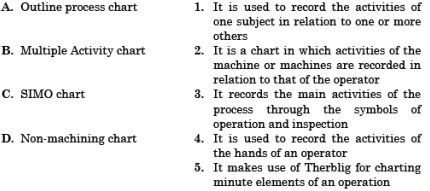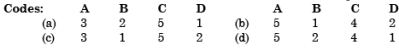Solution:
QUESTION: 29

A graphical representation of the coordinated activities (i.e., fundamental motions) of an operator's body members, on a common time scale is known as

Solution:
QUESTION: 30

Consider the following statements:

Method study is carried out to achieve
1. The most effective use of plant and equipment.
2. The most effective use of human efforts.
3. Evaluation of human work.

Which of the statements given above are correct?

Solution: